# 【C语言进阶学习】二、指针的进阶（2）

## 函数指针

&数组名 - - - 取出的是数组的地址

int Add(int x, int y)
{
return x + y;
}
int main()
{
return 0;
}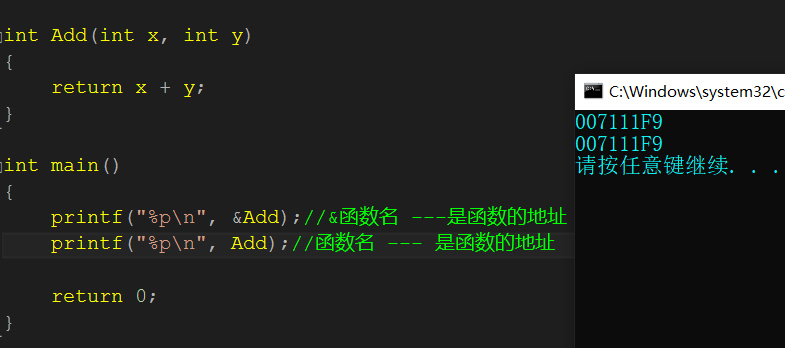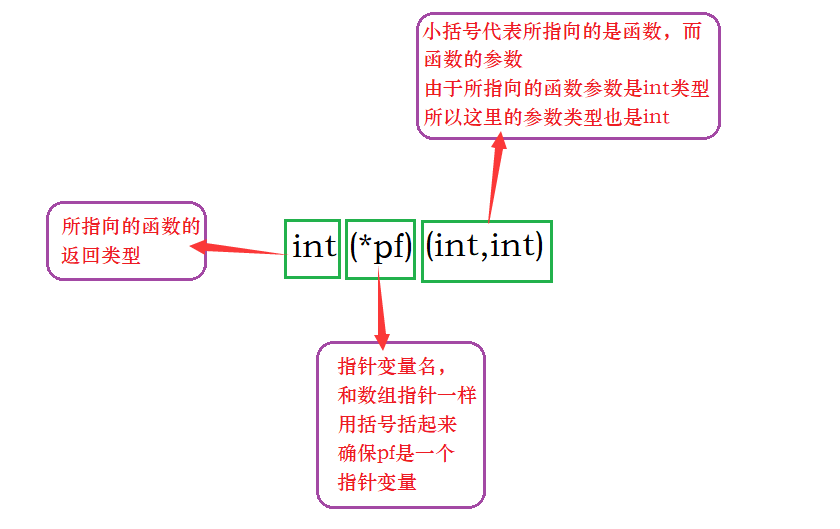.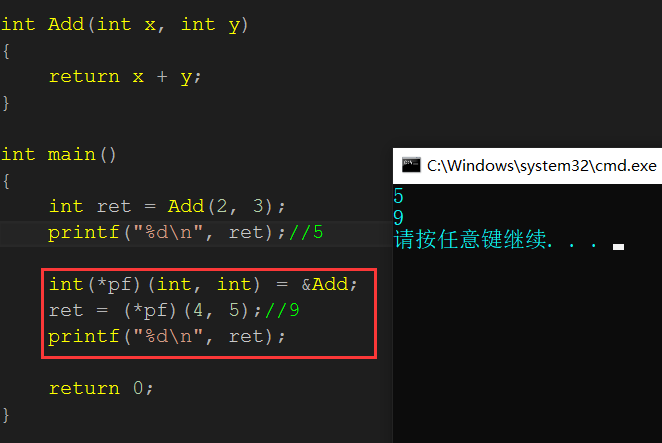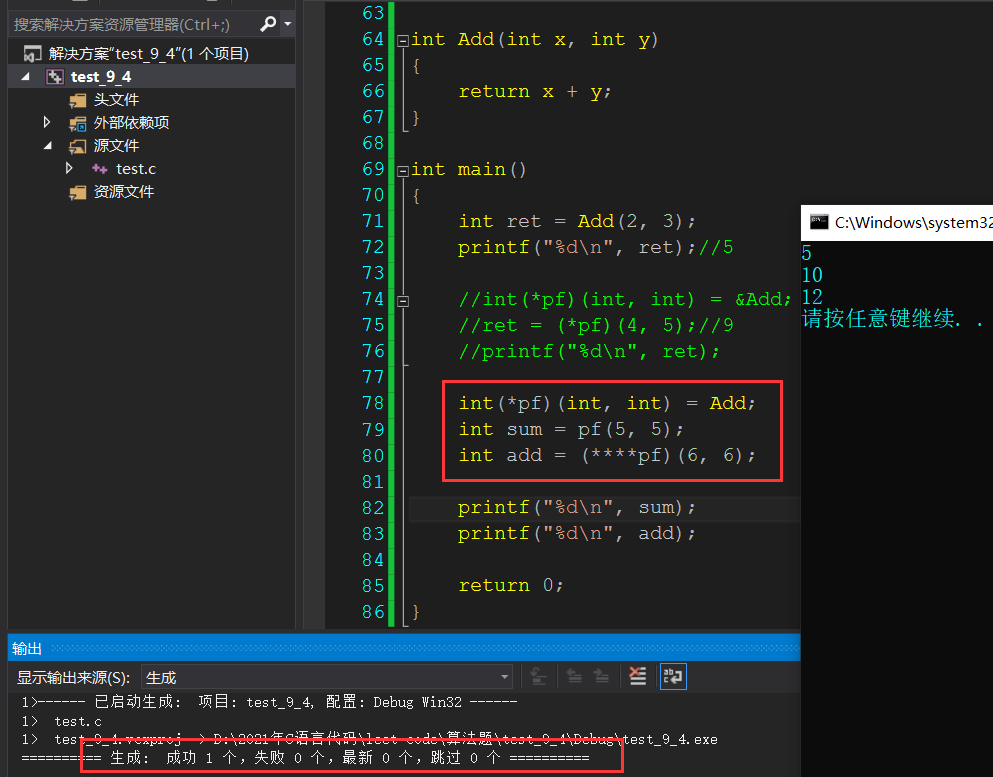//代码1
(*(void (*) ())0)();

//代码2
void(*signal(int, void(*)(int)))(int);


	( * ( void(*)() )0 )();
代码分析：
这个代码是一次函数调用
1.首先代码中把0强制类型转换为类型为void(*)()的一个函数的地址。
2.然后解引用0的地址，就是取0地址处的这个函数，被调用的函数是无参的，返回类型是void


void(* signal(int, void(*)(int) ) )(int);

signal函数有2个参数，第一个参数是int类型，第二个是void(*)(int)的函数指针类型
signal函数的返回类型依然是：void(*)(int)的函数指针类型


typedef void(*pfun_t)(int);
//typedef类型重定义关键字，将void(*)(int)类型的函数指针，重新命名为pfun_t
//所以代码2可以写为：
pfun_t signal(int,pfun_t);


## 函数指针数组

int* arr;
//整型指针数组---存放整型指针的数组
//该数组有10个元素，每个元素都是int*类型


#include <stdio.h>
//加法
int Add(int x, int y)//函数指针类型：int (*)(int, int)
{
return x + y;
}
//减法
int Sub(int x, int y)//函数指针类型：int (*)(int, int)
{
return x - y;
}
//乘法
int Mul(int x, int y)//函数指针类型：int (*)(int, int)
{
return x * y;
}
//除法
int Div(int x, int y)//函数指针类型：int (*)(int, int)
{
return x / y;
}

int main()
{
//pfArr就是一个函数指针的数组
int (* pfArr)(int, int) = {Add, Sub, Mul, Div};
//去掉pfArr剩下的int(*)(int,int)就是函数指针数组的元素的类型
return 0;
}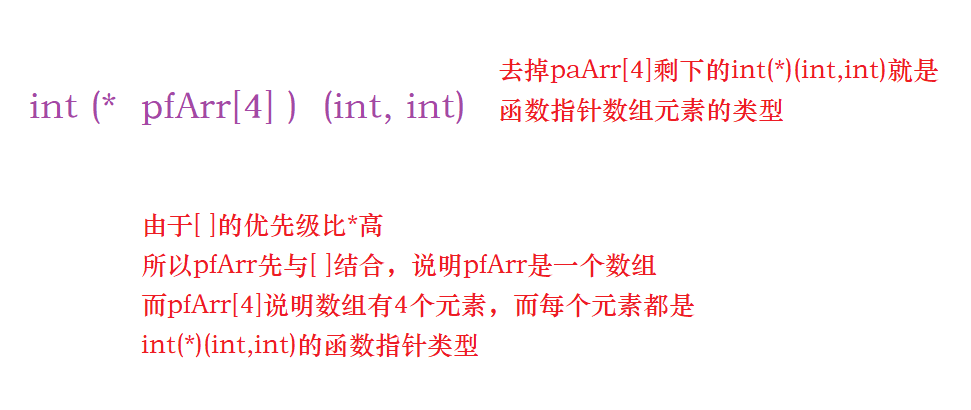#include <stdio.h>
{
printf("**********************************\n");
printf("******* 3.mul     4. div    ******\n");
printf("*******      0.exit         ******\n");
printf("**********************************\n");
}

//加法
int Add(int x, int y)//函数指针类型：int (*)(int, int)
{
return x + y;
}
//减法
int Sub(int x, int y)//函数指针类型：int (*)(int, int)
{
return x - y;
}
//乘法
int Mul(int x, int y)//函数指针类型：int (*)(int, int)
{
return x * y;
}
//除法
int Div(int x, int y)//函数指针类型：int (*)(int, int)
{
return x / y;
}

int main()
{
int input = 0;
int x = 0;
int y = 0;
int ret = 0;//接收结果
do
{
printf("请选择：>");
scanf("%d", &input);

switch (input)
{
case 1:
printf("请输入两个操作数：");
scanf("%d %d", &x, &y);
printf("ret = %d\n", ret);
break;
case 2:
printf("请输入两个操作数：");
scanf("%d %d", &x, &y);
ret = Sub(x, y);
printf("ret = %d\n", ret);
break;
case 3:
printf("请输入两个操作数：");
scanf("%d %d", &x, &y);
ret = Mul(x, y);
printf("ret = %d\n", ret);
break;
case 4:
printf("请输入两个操作数：");
scanf("%d %d", &x, &y);
ret = Div(x, y);
printf("ret = %d\n", ret);
break;
case 0:
printf("退出游戏\n");
break;
default:
printf("输入错误，请重新输入\n");
}
} while (input);
return 0;
}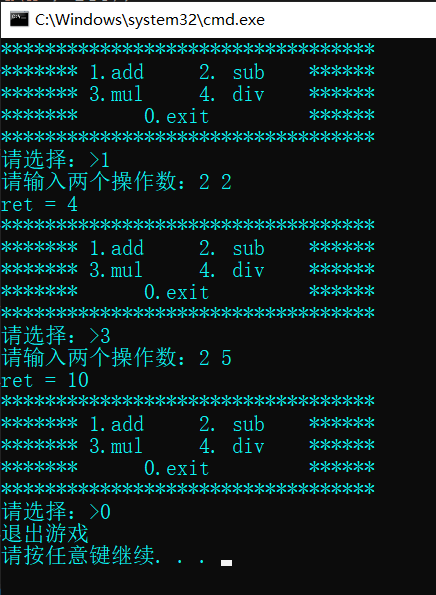void menu()
{
printf("**********************************\n");
printf("******* 3.mul     4. div    ******\n");
printf("*******      0.exit         ******\n");
printf("**********************************\n");
}
int Add(int x, int y)//int (*)(int, int)
{
return x + y;
}

int Sub(int x, int y)//int (*)(int, int)
{
return x - y;
}

int Mul(int x, int y)//int (*)(int, int)
{
return x * y;
}

int Div(int x, int y)//int (*)(int, int)
{
return x / y;
}

void Calc(int(*pf)(int, int))
{
int x = 0;
int y = 0;
int ret = 0;

printf("请输入2个操作数:>");
scanf("%d %d", &x, &y);
ret = pf(x, y);
printf("ret = %d\n", ret);
}

int main()
{
int input = 0;
do
{
printf("请选择:>");
scanf("%d", &input);
switch (input)
{
case 1:
break;
case 2:
Calc(Sub);
break;
case 3:
Calc(Mul);
break;
case 4:
Calc(Div);
break;
case 0:
printf("退出计算器\n");
break;
default:
printf("选择错误\n");
break;
}
} while (input);
return 0;
}


void menu()
{
printf("**********************************\n");
printf("******* 3.mul     4. div    ******\n");
printf("*******      0.exit         ******\n");
printf("**********************************\n");
}

//加法
int Add(int x, int y)//函数指针类型：int (*)(int, int)
{
return x + y;
}
//减法
int Sub(int x, int y)//函数指针类型：int (*)(int, int)
{
return x - y;
}
//乘法
int Mul(int x, int y)//函数指针类型：int (*)(int, int)
{
return x * y;
}
//除法
int Div(int x, int y)//函数指针类型：int (*)(int, int)
{
return x / y;
}

int main()
{
int input = 0;
int x = 0;
int y = 0;
int ret = 0;//接收结果
do
{
printf("请选择：>");
scanf("%d", &input);
//pfArr就是函数指针数组
int(*pfArr)(int, int) = { 0, Add, Sub, Mul, Div };
//下标  0   1    2    3    4
if (input == 0)
{
printf("退出计算器\n");
}
else if (input >= 1 && input <= 4)
{
printf("请输入两个操作数：");
scanf("%d %d", &x, &y);
ret = pfArr[input](x, y);
printf("ret = %d\n", ret);
}
else
{
printf("选择错误\n");
}
} while (input);
return 0;
}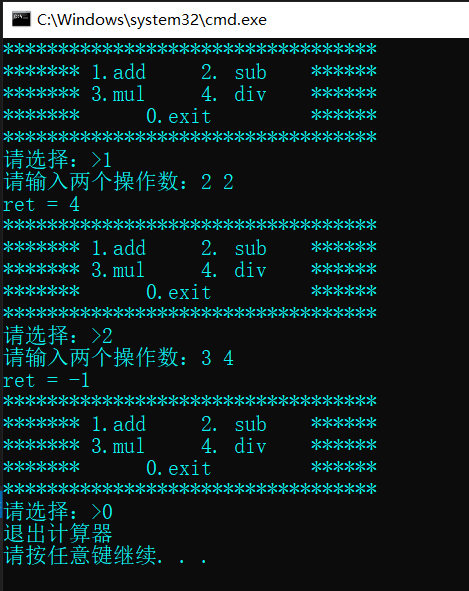## 指向函数指针数组的指针

#include <stdio.h>
{
return x + y;
}
int main()
{
int arr1;//整型数组
int(*p1) = &arr1;
//p1是指向一个整型数组的指针

int* arr2;//整型指针数组
int*(*p2) = &arr2;
//p2是一个指向整型指针数组的指针

//pf是一个函数指针
int(* pfArr)(int, int);
//pfArr是一个函数指针数组，该数组有五个元素，每个元素都是int(*)(int,int)类型的函数指针

int(* (*ppfArr) )(int,int) = &pfArr;
//ppfArr就是一个函数指针数组的指针
//它指向一个函数指针数组，该数组有五个元素，每个元素都是函数指针，类型为int(*)(int,int)
return 0;
}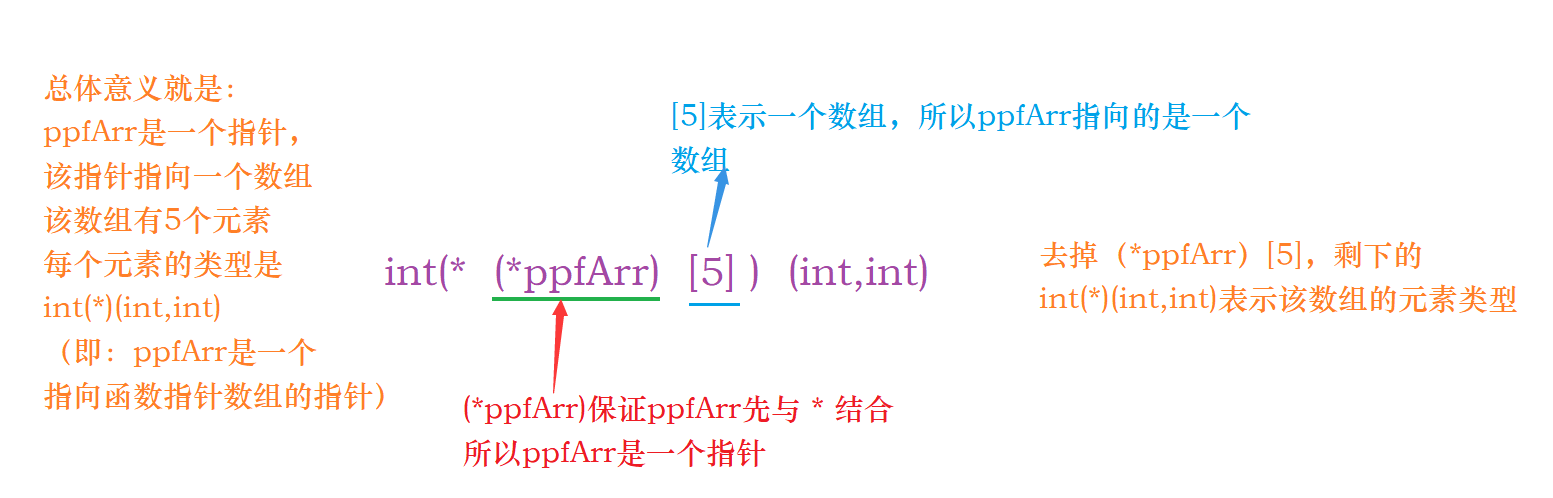## 回调函数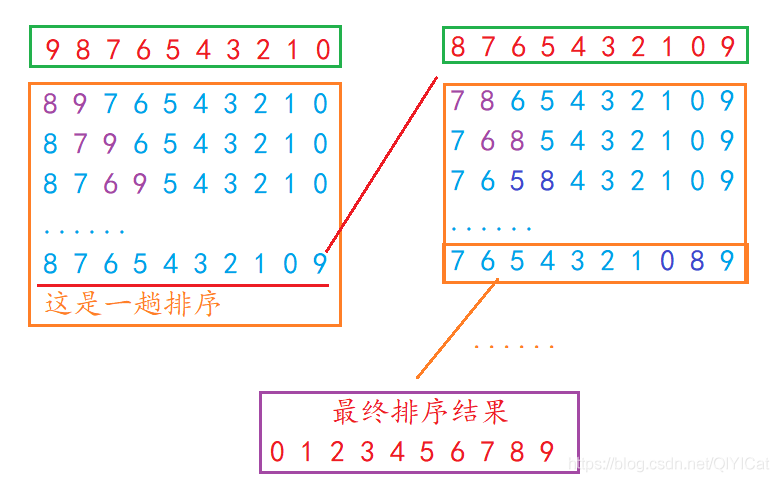……

#include <stdio.h>
void print(int* arr, int sz)
{
int i = 0;
for (i = 0; i < sz; i++)
{
printf("%d ", *(arr + i));
}
printf("\n");
}
void bubble_sort(int* arr, int sz)
{
int i = 0;
for (i = 0; i < sz - 1; i++)//确定冒泡排序的趟数
{
int j = 0;
int flag = 1;//假设本趟已经有序了

for (j = 0; j < sz - 1 - i; j++)//确定每一趟两两比较的次数
{
if (*(arr + j) > *(arr + j + 1))
{
int tmp = *(arr + j);
*(arr + j) = *(arr + j + 1);
*(arr + j + 1) = tmp;
flag = 0;//本趟无序
}
}
if (flag == 1)
{
break;
}
}
}
int main()
{
int arr = { 9, 8, 7, 6, 5, 4, 3, 2, 1, 0 };
int sz = sizeof(arr) / sizeof(arr);

printf("排序前：\n");
print(arr, sz);

//通过冒泡法，进行升序排列
bubble_sort(arr, sz);

printf("排序后：\n");
print(arr, sz);
return 0;
}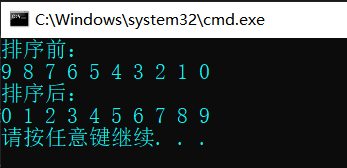qsort - - - 使用快速排序的方法
qsort库函数的相关信息：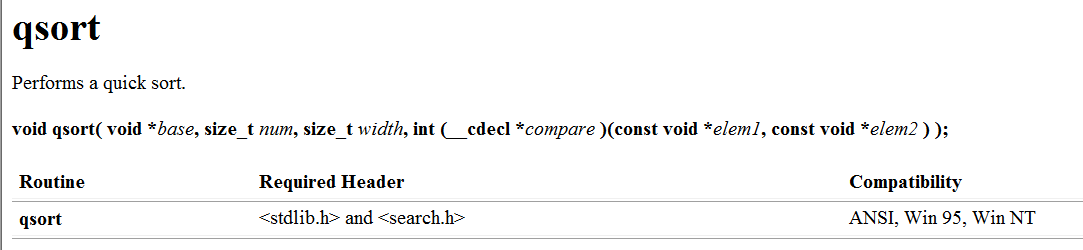void qsort(
void* base,//待排序目标的起始位置
size_t num,//待排序的元素个数
size_t width,//一个元素的大小，单位是字节
int(* cmp)(const void* e1, const void* e2)
//函数指针，排序所使用的比较函数
//不同类型的数据比较的方法是不一样的，所以需要将比较方法写成函数传递给qsort
//函数指针，指针指向的参数有两个，参数类型都是const void*,函数返回类型是int
)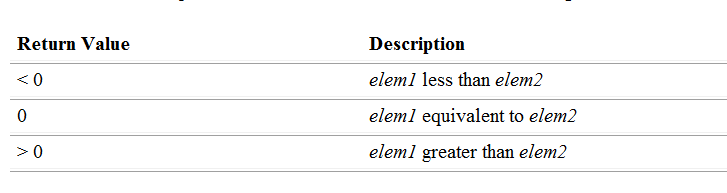void*类型指针使用注意类项：

1. void* 类型指针不能进行解引用操作的。
（原因：指针的类型决定了指针解引用操作时访问字节的个数，如果指针类型是void *，无类型指针，那么就无法得知该指针解引用访问多少个字节数。）
2. void* 类型指针不能进行++ / ±整数运算的操作。
（原因：指针类型的意义决定了指针 + -整数运算的步长，如果指针类型是void *无类型指针，那么就无法得知该指针 + -的步长到底是多少个字节。）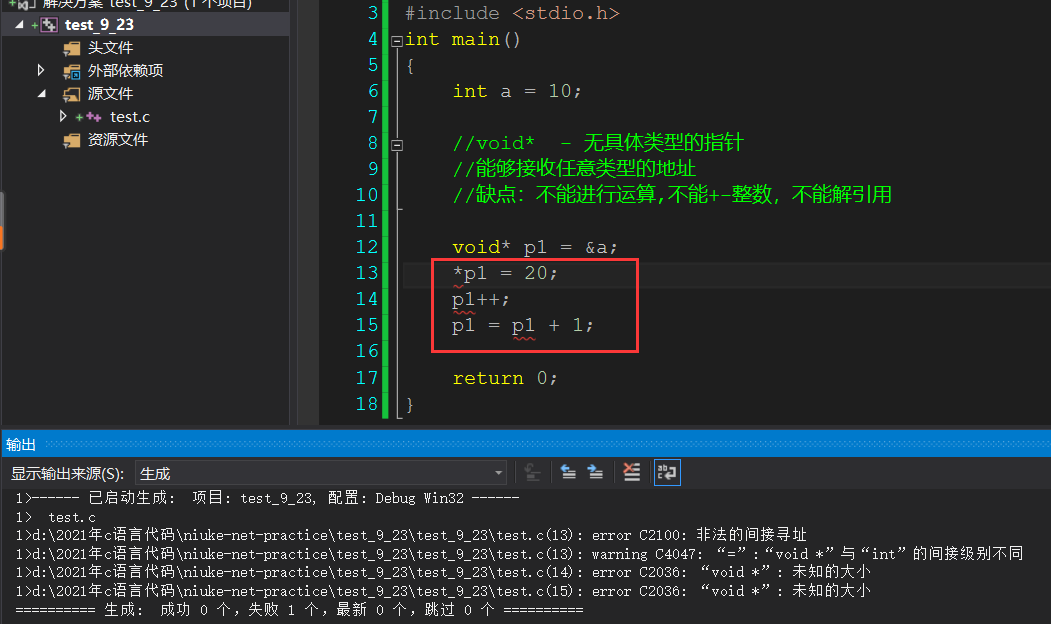（1）下面我们来试着用qsort排序一下整型数组，熟悉qsort的使用：

#include <stdio.h>
#include <stdlib.h>
#include <string.h>
//比较2个整型的函数
//为了调用qsort，需要将比较函数传给qsort
//按照qsort函数参数的类型，写出需要使用的比较函数
int cmp_int(const void* e1, const void*e2)
{
return *(int*)e1 - *(int*)e2;//升序
//return *(int*)e2 - *(int*)e1;//降序

}

void print_arr(int arr[], int sz)
{
int i = 0;
for (i = 0; i < sz; i++)
{
printf("%d ", arr[i]);
}
}

void test1()
{
int arr[] = { 10, 8, 9, 7, 5, 3, 4, 2, 1, 6 };
int sz = sizeof(arr) / sizeof(arr);
qsort(arr, sz, sizeof(arr), cmp_int);
//打印
print_arr(arr, sz);
}
int main()
{
//排序整型数组
test1();
return 0;
}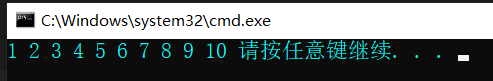（2）利用qsort排序结构体数据

#include <stdio.h>
#include <stdlib.h>
#include <string.h>
struct stu
{
char name;
int age;
};

int cmp_by_name(const void*e1,const void*e2)
{
//字符串比较需要用到字符串比较库函数strcmp
return strcmp(((struct stu*)e1)->name, ((struct stu*)e2)->name);
}

int cmp_by_age(const void*e1, const void*e2)
{
return ((struct stu*)e1)->age - ((struct stu*)e2)->age;
}

void print_s(struct stu s[], int sz)
{
int i = 0;
for (i = 0; i < sz; i++)
{
printf("%s,%d\n", s[i].name, s[i].age);
}
printf("\n");
}

void test2()
{
struct stu s = { { "zhangsan", 15 }, { "lisi", 10 }, { "wangwu", 30 } };
int sz = sizeof(s) / sizeof(s);
int i = 0;

qsort(s, sz, sizeof(s), cmp_by_name);//按名字来排

//打印
printf("按名字排序：\n");
print_s(s, sz);

qsort(s, sz, sizeof(s), cmp_by_age);//按名字来排

//打印
printf("按年龄排序：\n");
print_s(s, sz);
}

int main()
{
test2();//排序结构体
return 0;
}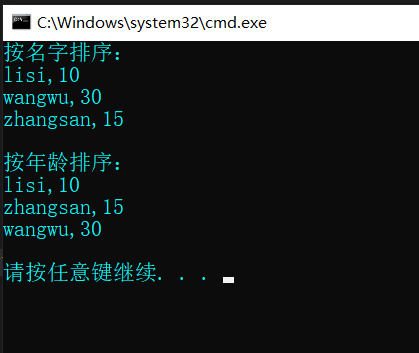（3）利用qsort排序浮点型数据：

#include <stdio.h>
#include <stdlib.h>
#include <string.h>
int cmp_float(const void* e1, const void* e2)
{
//强制类型转换成int类型---float类型转换为int类型会造成精确度缺失
return (int)(*(float*)e1 - *(float*)e2);
}

void print_float(float arr2[], int sz)
{
int i = 0;
for (i = 0; i < sz; i++)
{
printf("%.1f ",arr2[i]);
}
printf("\n");
}
void test3()
{
float arr2[] = { 4.0, 5.0, 1.0, 2.0, 3.0, 9.0 };
int sz = sizeof(arr2) / sizeof(arr2);
int i = 0;

qsort(arr2, sz, sizeof(arr2), cmp_float);

//打印
print_float(arr2, sz);
}

int main()
{
test3();//排序浮点型数组
return 0;
}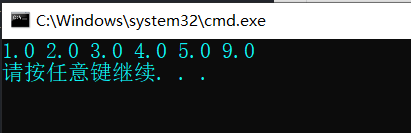#include <stdio.h>
#include <stdlib.h>
#include <string.h>
struct stu
{
char name;
int age;
};

int cmp_int(const void*e1, const void*e2)
{
return (*(int*)e1) - (*(int*)e2);
}

int cmp_by_name(const void*e1,const void*e2)
{
return strcmp(((struct stu*)e1)->name, ((struct stu*)e2)->name);
}

int cmp_by_age(const void*e1, const void*e2)
{
return ((struct stu*)e1)->age - ((struct stu*)e2)->age;
}
//交换函数
void Swap(char* buf1, char* buf2, int width)
{
int i = 0;
for (i = 0; i < width; i++)//一个一个字节进行交换
{
char tmp = *buf1;
*buf1 = *buf2;
*buf2 = tmp;
buf1++;
buf2++;
}
}
//使用回调函数实现一个通用的冒泡排序函数
void BubbleSort(void* base, size_t num, size_t width, int (*cmp)(const void*e1, const void*e2))
{
size_t i = 0;
//趟数
for (i = 0; i < num - 1; i++)
{
//比较的对数
size_t j = 0;
for (j = 0; j < num - 1 - i; j++)
{
if (cmp((char*)base+j*width, (char*)base+(j+1)*width)>0)
{
//交换
Swap((char*)base + j * width, (char*)base + (j + 1) * width, width);//只有一个一个字节才可以完成交换
}
}
}
}
//测试自定义的BubbleSort()排序整型数组
void test3()
{
int arr[] = { 9,8,7,6,5,4,3,2,1,0 };
int sz = sizeof(arr) / sizeof(arr);

BubbleSort(arr, sz, sizeof(arr), cmp_int);

//打印
int i = 0;
for (i = 0; i < sz; i++)
{
printf("%d ", arr[i]);
}
printf("\n");
}
//测试自定义的BubbleSort() 排序结构体
void test4()
{
struct stu s = { {"zhangsan", 15}, {"lisi", 30},{"wangwu", 10} };
int sz = sizeof(s) / sizeof(s);
//按照名字排序
BubbleSort(s, sz, sizeof(s), cmp_by_name);

//打印
int i = 0;
printf("\n按名字排序：\n");
for (i = 0; i < sz; i++)
{
printf("%s,%d\n", s[i].name, s[i].age);
}
printf("\n");

//按照年龄来排序
BubbleSort(s, sz, sizeof(s), cmp_by_age);

//打印
printf("按年龄排序：\n");
for (i = 0; i < sz; i++)
{
printf("%s,%d\n", s[i].name, s[i].age);
}
printf("\n");

}
int main()
{
//测试自定义的BubbleSort();
test3();//测试排序整型数组

test4();//排序结构体数据

return 0;
}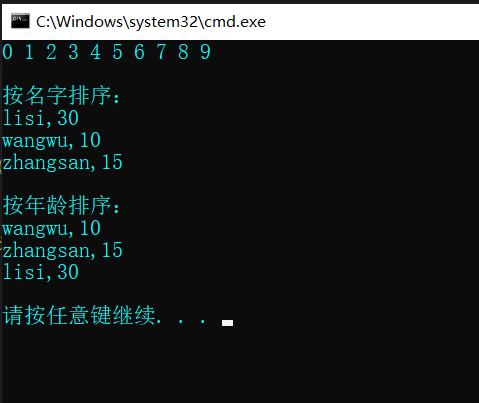12-17
10-09
10-12
08-033821
10-02
07-12638
01-19
12-1821
03-08656
10-12
08-05195
04-11
06-24
07-23
09-27450

### “相关推荐”对你有帮助么？

•非常没帮助
•没帮助
•一般
•有帮助
•非常有帮助被折叠的  条评论 为什么被折叠?到【灌水乐园】发言Clumsy、笨拙

¥2 ¥4 ¥6 ¥10 ¥20余额支付 (余额：-- )扫码支付获取中扫码支付点击重新获取扫码支付1.余额是钱包充值的虚拟货币，按照1:1的比例进行支付金额的抵扣。
2.余额无法直接购买下载，可以购买VIP、C币套餐、付费专栏及课程。余额充值